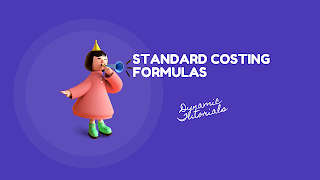# CMA CA CS Inter/Final: Standard Costing Formula | Strategic Cost Management | CMA Important Topics### STANDARD COSTING FORUMULASFor CMA, CA, CS, B.Com, M.Com, BBA Exam

Key Points (Tricks) to be remembered while solving practical problems:
SR = Standard Rate (Given)
AR = Actual Rate (Given)
SQ = Standard Quantity (Based on Actual output = SQ*AY/SY)
AQ = Actual Quantity (Given)
RSQ = Revised Standard Quantity (SQ*Actual Input/Standard Input)
AY = Actual Yield (given)
SY = Standard Yield (Actual input – Standard loss)

## Material Variance

1. Material Cost Variance (MCV) = SRXSQ – ARXAQ
2. Material Price Variance (MPV) = AQ (SR – AR)
3. Material Usage/Quantity Variance (MUV) = SR (SQ – AQ)
4. Material Mix Variance (MMV) = SR (RSQ – AQ)
5. Material Yield Variance (MYV) = SR (AY – SY)
Verification: MCV = MPV+MUV; MUV = MMV+MYV

## Labour Variance

1. Labour Cost Variance (LCV) = SRXSH – ARXAH [Actual hours including Idle time]
2. Labour Rate of Pay Variance (LRPV) = AH (SR – AR)
3. Total Labour efficiency variance (TLEV) = SR (SH – AH) [Actual hours including idle time]
4. Labour Efficiency/Time Variance (LEV/LTV) = SR (SH – AH) [Actual Hours deducting idle time]
4. Labour Revised Efficiency Variance (LREV) = (SH – RSH) X Standard Rate
4. Labour Mix Variance (LMV) = SR (RSH – AH) [RSH is calculated in the same way as in the case of material]
5. Labour Yield Variance (LYV) = SR (AY – SY)
6. Labour Idle Time Variance = SR X Idle Hours
Key points in labour variance:
1. Standard Gang Hours = Actual output/Standard output per gang hours
2. Actual data are always given.
3. Idle time may be normal or abnormal. It does not affect formula.
4. Verification: LCV = LRPV+LEV + Idle Time Variance. LEV = LMV+LREV
5. After TLEV, all calculations are made on the basis of actual hours worked.
6. In case of change in the percentage of skilled and unskilled labour, only standard is to be adjusted. Actual figures are given after all adjustments.

## Fixed overhead variance

1. Fixed overhead cost variance = Fixed overhead recovered (AO*SR) – Actual fixed overhead (AO*AR)
2. Fixed overhead expenditure variance = Actual fixed overhead (AO*AR) – Budgeted fixed overheads (BO*BR)
3. Fixed overhead volume variance = Budgeted fixed overhead (BO*BR)– Fixed overhead recovered (AO*SR)
4. Fixed overhead Capacity variance = Budgeted fixed overhead (BO*BR) – Standard fixed overheads (SO*SR)
5. Idle time variance = Idle hours X standard fixed overhead rate per hour
6. Fixed overhead efficiency variance =Standard fixed overhead  - Fixed Overhead recovered
7. Fixed Overhead Calendar variance = Excess/Deficit hours worked X Standard fixed overhead rate per hour
(Here, AO = Actual Output - Given; BO = Budgeted Output - Given; SO = Standard Output – Always Based on actual working days;)
Verification: Fixed overhead cost variance = Expenditure variance + volume variance
Fixed overhead volume variance = Capacity variance + efficiency variance + Idle time variance + calendar variance.

## Variable overhead variance

1. Variable overhead cost variance = Variable overhead recovered (SO*SR) – Actual Variable overhead (AO*AR)
2. Fixed overhead expenditure variance = Actual fixed overhead (AO*AR) – Standard variable overheads (SO*BR)
3. Fixed overhead efficiency variance =Standard variable overhead  - variable Overhead recovered

## Activity Ratios

1.       Efficiency Ratio = Standard Hours/Actual hours x 100
2.       Activity Ratio = Standard Hours for Actual Work/Budgeted standard hours x 100
3.       Calendar Ratio = Available Working Days/Budgeted Working Days x 100
4.       Capacity Usage Ratio = Budgeted Hours/Maximum Possible Hours in Budget Period x 100
5.       Capacity Utilisation Ratio = Actual Hours/Budgeted Hours x 100
6.       Idle Time Ratio = Ideal Time Hours/Budgeted Hours x 10

## Sales variance (On the basis of value and margin)

### Sales Variance (On the basis of Value)

1. Sales Value Variance = (Actual Sales - Budgeted Sales)
2. Sales Price Variance = (Actual Price - Standard Price) x Actual unit sold.
3. Sales Volume Variance = (Actual Units - Budgeted Units) x Std. Price.
4. Sales Mix Variance = (Actual Units  - Total Actual units in Std. Ratio) x Std. Price.
5. Sales Quantity Variance = (Total Actual units in Std. Ratio - Budgeted units) x Std. Price.

### Sales variance (On the basis of margin when both sale price and cost is given)

1. Sales Margin / Profit / Variance = Actual Profit - Budgeted Profit.
2. Sales margin Price Variance = Actual units (Actual Profit – Budgeted Profit)
3. Sales Margin volume variance = (Actual quantity - Budgeted quantity) standard margin
4. Sales Margin mix variance   = (Actual quantity - total quantity standard ratio) standard margin
5. Sales margin quantity variance = (Total qty. in Std  Ratio - budgeted quantity) x Std. Margin
6. Market Share variance = (Actual sales units – budgeted share of actual market ) std margin p.u.
7. Market Size variance  = (budgeted share of actual market – budgeted sales units) std margin p.u.